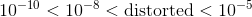## Example Questions

### Example Question #11 : How To Find Synthesis Of Data In Physics

Laura is performing an experiment with a 5kg weight tied to a 3m rope tied to the ceiling as shown: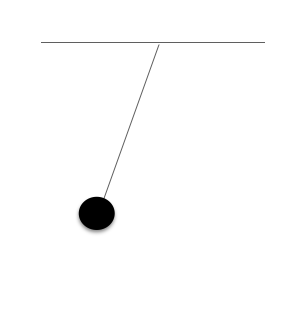Laura drops the weight and allows it to swing freely. She measures how long it takes for the weight to return to its original position (assume no forces outside of gravity are acting upon the pendulum). This is also called one oscillation.

Experiment 1:

Laura created the following table for her first measurement of the pendulum's oscillations.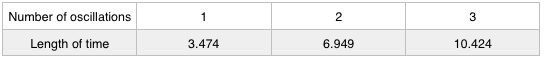Experiment 2:

Laura performed the experiment again, this time using a 6kg weight.Experiment 3:

Laura performed the experiment again, this time using a 3kg weight and a 5m rope.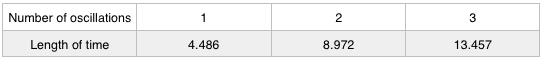If Laura stopped experiment 3 after 10 seconds, how many oscillations would the pendulum have gone through?

2.23

2.5

2

3

2.23

Explanation:

Since each oscillation lasts 4.486 seconds,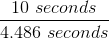gives us the answer of 2.23 oscillations.

### Example Question #12 : How To Find Synthesis Of Data In Physics

Mary is performing an experiment involving the electromagnetic spectrum. She observes several different types of waves and records their wavelength, frequency, and speed.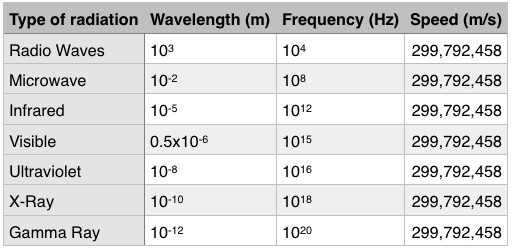A new type of radiation is discovered on the electromagnetic spectrum that has a higher frequency than gamma rays. What would its speed most likely be?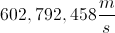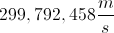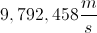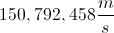Explanation:

Notice that all of the waves, regardless of their frequencies move at the same speed: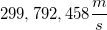. The newly discovered wave would also move at.

### Example Question #81 : Physics

Mary is performing an experiment involving the electromagnetic spectrum. She observes several different types of waves and records their wavelength, frequency, and speed.A new type of radiation is discovered with wavelength measurements between those of microwaves and infrared radiation. What is the most likely value of its frequency?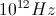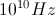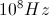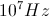Explanation:

If the new radiation has a wavelength between the wavelengths of microwaves and infrared waves, then it must have a frequency between the frequencies of microwaves and infrared waves as well. Following the trends in the table, we can set up the flowing inequalities.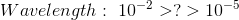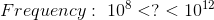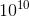is the only answer choice that satisfies the inequality for frequency.

### Example Question #14 : How To Find Synthesis Of Data In Physics

Mary is performing an experiment involving the electromagnetic spectrum. She observes several different types of waves and records their wavelength, frequency, and speed.A soccer field is approximately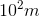long. Which wave has a wavelength closest to the length of a soccer field?

Visible light

Microwaves

Infrared waves

Explanation:

Radio waves have a wavelength of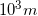, which is the closest to the soccer field's length of. All of the other waves have wavelengths that are much smaller, due to the negative exponents.

### Example Question #15 : How To Find Synthesis Of Data In Physics

Mary is performing an experiment involving the electromagnetic spectrum. She observes several different types of waves and records their wavelength, frequency, and speed.A water molecule is approximately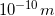in diameter. Which wave has a wavelength closest to this length?

Microwaves

Gamma rays

X-rays

X-rays

Explanation:

X-rays have a wavelength of, which is exactly the same size as the water molecule mentioned in the problem.

Radio waves and microwaves have wavelengths that are larger than the diameter of the water molecule, and gamma rays have a wavelength that is smaller.

### Example Question #16 : How To Find Synthesis Of Data In Physics

Mary is performing an experiment involving the electromagnetic spectrum. She observes several different types of waves and records their wavelength, frequency, and speed.With which of the following variables is wavelength inversely proportional?

Speed

Direction

Frequency

Size

Frequency

Explanation:

The trends in the table, from top to bottom, are a decrease in wavelength, an increase in frequency, and no change in speed. Based on these trends, we can see that as wavelength decreases, frequency increases. This type of trend describes an inverse proportionality.

### Example Question #82 : Physics

Mary is performing an experiment involving the electromagnetic spectrum. She observes several different types of waves and records their wavelength, frequency, and speed.The Earth's atmosphere prevents certain wavelengths on the electromagnetic spectrum from reaching the Earth's surface. If anything smaller than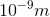is blocked, then which of the following rays would not reach the Earth's surface?

Visible Light

Gamma rays

Ultraviolet light

Gamma rays

Explanation:

The question states that any wavelengths less thanare blocked and do not reach the surface.

The only answer choice with a wavelength smaller thanis gamma rays, which have a length of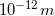.

### Example Question #1201 : Act Science

Mary is performing an experiment involving the electromagnetic spectrum. She observes several different types of waves and records their wavelength, frequency, and speed.An AM radio wave has a frequency of approximately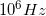. What would be a possible wavelength for this wave?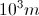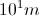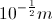Explanation:

An AM Radio wave has a frequency in the middle between the given radio wave frequency and the given microwave frequency.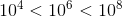We should assume the wavelength will also be between their two values. The only answer choice that satisfies this requirement is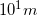.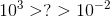### Example Question #1201 : Act Science

Mary is performing an experiment involving the electromagnetic spectrum. She observes several different types of waves and records their wavelength, frequency, and speed.Mary realizes that all of the waves on the electromagnetic spectrum are moving at the speed of light. Which of these answer choices is a possible conclusion for this experiment?

There are the different possible sizes, shapes, and speeds that light can take

Light operates as both a particle and a wave

Light operates purely as a wave with a constant frequency

These are the different frequencies and wavelengths of light

These are the different frequencies and wavelengths of light

Explanation:

Based upon the experiment, Mary can assume that all of these waves are operating at the speed of light, but with different frequencies and wavelengths.

The other choices are incorrect as the experiment does not mention light being a particle. The experiment shows that these waves do not have a constant frequency. The problem does not talk about a "shape" of light.

### Example Question #81 : Physics

Mary is performing an experiment involving the electromagnetic spectrum. She observes several different types of waves and records their wavelength, frequency, and speed.The Earth's atmosphere allows types of radiation to enter the atmosphere, and blocks others. It allows in wavelengths from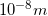to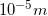, but creates some distortion. Which type of radiation would not potentially be affected by the distortion?

X-rays

Visible light

Infrared

Ultraviolet